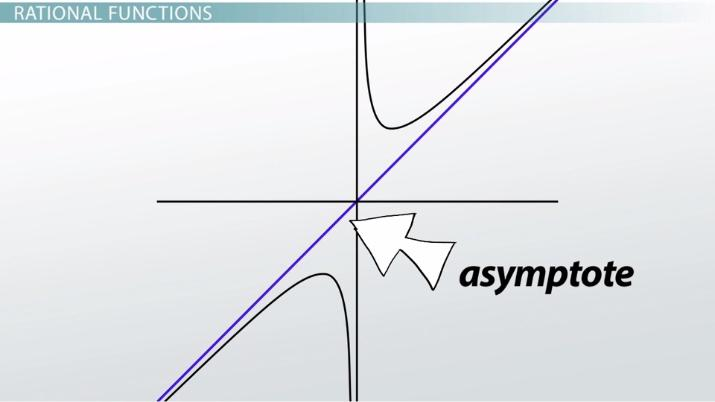Finding Slant Asymptotes of Rational Functions

Lesson Transcript
Instructor: Yuanxin (Amy) Yang Alcocer

Amy has a master's degree in secondary education and has been teaching math for over 9 years. Amy has worked with students at all levels from those with special needs to those that are gifted.

Slant asymptotes occur when the denominator polynomial degree is less than the degree of the numerator polynomial by one degree. Learn about finding the slant asymptotes of rational functions with examples. Updated: 11/03/2021

Rational Functions

1. f (x) = x / x^2

2. f (x) = 2x^4 - 3x^2 / 3x^4 + 5x - 9

The first rational function has a horizontal asymptote of y = 0 because the degree of the denominator polynomial is greater than the degree of the numerator polynomial. Also, this function has a vertical asymptote of x = 0 because, if we set the denominator equal to 0 and solve, we will get x = 0. The second rational function has a horizontal asymptote of y = 2/3 because the degree of both the numerator and denominator polynomial are the same. We got y = 2/3 by isolating the leading coefficient of the numerator polynomial and the leading coefficient of the denominator polynomial.

We know how to find vertical and horizontal asymptotes. But what happens when the degree of our numerator polynomial is greater than the degree of our denominator polynomial by 1? For example, if you see a function like this.

y = x^2 + 3x +2 / x - 2

What happens then? We get what is called a slant asymptote, an asymptote that is neither horizontal nor vertical but slanted.An error occurred trying to load this video.

Try refreshing the page, or contact customer support.

Coming up next: Expressions of Rational Functions

You're on a roll. Keep up the good work!

Replay
Your next lesson will play in 10 seconds
• 0:02 Rational Functions
• 2:41 Finding the Slant Asymptote
• 3:56 Example 1
• 5:05 Example 2
• 6:34 Lesson Summary
Save Save

Want to watch this again later?

Timeline
Autoplay
Autoplay
Speed Speed

Finding the Slant Asymptote

You know from your previous understanding of graphs that a straight slanted line will have an equation in the form of y = mx + b, where m is the slope and b is the y-intercept. The question now becomes, how do you find this slant asymptote and its equation from your rational function?

As it turns out, it is a fairly straightforward process. It does require that you know how to divide polynomials. To find a slant asymptote when the degree of the numerator polynomial is exactly one more than the degree of the denominator polynomial, you need to perform the division that is shown by the rational function. The slant asymptote is then your answer, not including the remainder if there is one. Remember that dividing polynomials is very similar to dividing large numbers. We take each term with its coefficient and variable and treat it like a place value when dividing numbers. For example, the constant is treated like our ones place, the term with the x variable is treated like the tens place, the term with the x^2 variable is treated like the hundreds place, and so on.

Let's look at a couple of examples to see this polynomial division in action.

Example 1

Find the slant asymptote of this rational function.

f (x) = x^3 - 5x / x^2 + 1

To unlock this lesson you must be a Study.com Member.

Register to view this lesson

Are you a student or a teacher?